Name:    2nd Grade Math Basic Test 6

Multiple Choice
Identify the choice that best completes the statement or answers the question.

1.

Number Sense and Numeration – Whole Numbers – RIT 161 – 170
What are the missing numbers?
____, 60, 61, 62, 63, 64, 65, 66, 67, 68, 69, ____
 a. 55, 75 d. 59, 70 b. 56, 72 e. 45, 70 c. 49, 80

2.

What number is 10 less than 58?
 a. 38 d. 68 b. 48 e. 57 c. 50

3.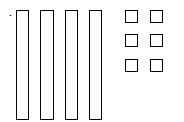What number does this picture above show
 a. 46 d. 36 b. 53 e. 16 c. 22

4.

Number Sense and Numeration – Fractions – RIT 171 – 180Which shows 1/2 of this candy bar?
 a.d.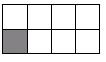b.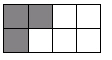e.c.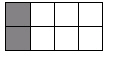5.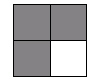What fraction of this square is shaded?
 a. 1/3 d. 3/4 b. 1/4 e. 3/3 c. 4/3

6.

Number Sense and Numeration – Ordering, Equalities, and Inequalities –RIT 171 – 180
Which number statement is correct?
 a. 134  <  133 d. 184  <   182 b. 468  >  486 e. 90   >  100 c. 236  >  234

7.

Number Sense and Numeration – Place Value – RIT 171 – 180
What is 3,406 written in expanded form?
 a. 3,000 + 40 + 6 d. 3,000 + 400 + 6 b. 300 + 400 + 60 e. 300 + 40 + 6 c. 3,000 + 400 + 60

8.

Number Sense and Numeration – Whole Numbers – RIT 171 – 180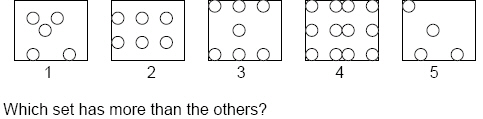a. 5 d. 2 b. 4 e. 1 c. 3

9.

16, 26, ____, 46, 56, 66, ____, 86
What are the missing numbers?
 a. 27, 67 d. 28, 68 b. 30, 40 e. 40, 80 c. 36, 76

10.

Which is a true statement?
 a. 86  >  72 d. 41  <  40 b. 96  >  97 e. 19  =  29 c. 63  >  64

11.

Which of these are only odd numbers?
 a. 2, 4, 6, 8 d. 11, 14, 16, 19 b. 12, 14, 16, 18 e. 17, 19, 22, 24 c. 11, 13, 15, 17

12.

____, 6, 8, ____, 12, 14, ____, ____, 20
What are the missing numbers?
 a. 5, 9, 15, 16 d. 3, 9, 15, 18 b. 4, 10, 16, 18 e. 1, 7, 18, 19 c. 5, 10, 16, 18

13.

Problem Solving – RIT 161 – 170
There are 5 blue bears and 4 red bears. How many bears are there in all?
 a. 6 d. 7 b. 10 e. 1 c. 9

14.

Statistics/Probability and Graphing – Graphing – RIT 151 – 160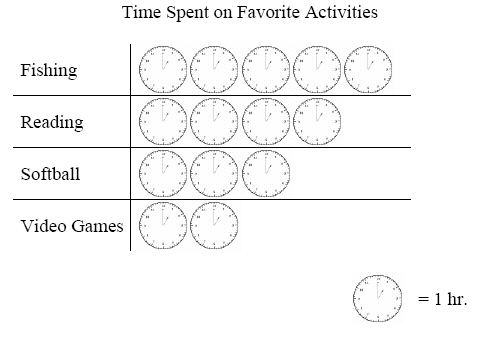How much more time is spent on fishing than reading?
 a. 1 d. 4 b. 2 e. 5 c. 3

15.

Statistics/Probability and Graphing – Graphing – RIT 161 – 170a. 6 d. 3 b. 5 e. 7 c. 4